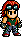# Wang Jinrei/Tekken Tag Tournament Movelist

Wang Jinrei/TTT Movelist is a list of the moves that Wang Jinrei can perform in Tekken Tag Tournament, a non-canonical video game in the Tekken fighting game series.Looks like Hwoarang has run off and made this page, Wang Jinrei/Tekken Tag Tournament Movelist, without telling Baek Doo San and hasn't done it right!Please fix it by re-formatting this page to follow it's [[Template:{{{1}}}|template]].

## §Moves List

1. Mountain Splitter: Square + Triangle.
2. G-Clef Cannon 1: Square Square Square.
3. G-Clef Cannon 2: Southeast + Square Square.
4. Slow Power Punch: Southeast + Triangle.
5. Slow Power Punch Combo: Square (must hit during Slow Power Punch.).
6. Snap Kick: Southeast + X.
7. Power Punch: East + Triangle.
8. Rising Heaven Kick: East + Circle.
9. Tiger Mountain: Southwest + Circle Triangle.
10. False Lift: Southwest + Triangle.
11. Double Palm Thrust: East + Square + Triangle.
12. Dragon Power Punch: West + Square + Triangle.
13. Medium Power Punch: Southeast + Square + Triangle.
14. Foot Stomp: Northeast (hold down) + X + Circle.
15. Spin Behind: Triangle West (must hit.).
16. Sweep: South + Circle.
17. Sweep to Razor's Edge: South + Circle Square.
18. Sweep to High Kick: South + Circle Circle.
19. Sweep to Low Kick: South + Circle South (hold down) + Circle.
20. Triple Spin Razor: Circle Circle Square.
21. Triple Spin Kicks: Circle Circle Circle.
22. Triple Spin Low: Circle Circle South (hold down) + Circle.
23. Heavy Uppercut: West West (hold down) + Square.
24. Parting Sweep: Circle (during sidestep.).
25. Horse Tamer: Square + Triangle (during sidestep.).
26. Heavy Power Punch: South Southeast East + Triangle.
27. Spinning Sweep: Southeast + Circle (while crouching.).
28. Spinning Sweep Combo: X (during Spinning Sweep.).
29. Skyscraper Kick: Circle (while rising.).
30. Tequila Sunrise: Triangle (while rising.).
31. Tequila Sunrise Combo: Triangle Square Square (while rising.).
32. Supercharger: X + Square + Triangle + Circle.
33. 10 Hit Combo: Triangle Square Square Triangle X X X Circle Circle Square.
34. Headlock Toss: (Approach enemy) Square + X.
35. Body Slam: (Approach enemy) Triangle + Circle.
36. Crushing the Dragon: (From the left) Square + X (or Triangle + Circle.).
37. Golden Mountain: (From the right) Square + X (or Triangle + Circle.).
38. Reverse Neck Throw: (From behind) Square + X (or Triangle + Circle.).
39. Reverse Throw: (Back towards enemy) X + Square (or Triangle + Circle.).
40. Behind the Back Suplex: (During Spin Behind) Square + Triangle.
41. Waning Moon: (Approach enemy) Southeast Southeast (hold down) + Triangle + Circle. Waning Moon
42. Dragon Thrust: (Approach enemy) Southeast (hold down) + Circle + X + Square.
43. Attack Reversal: (During enemy's attack) West + Square + X (or Triangle + Circle.).